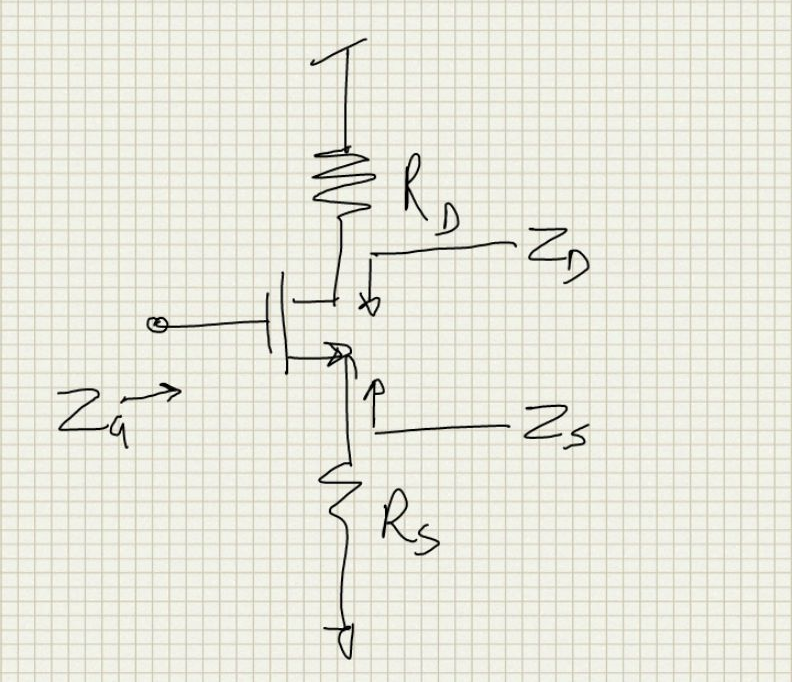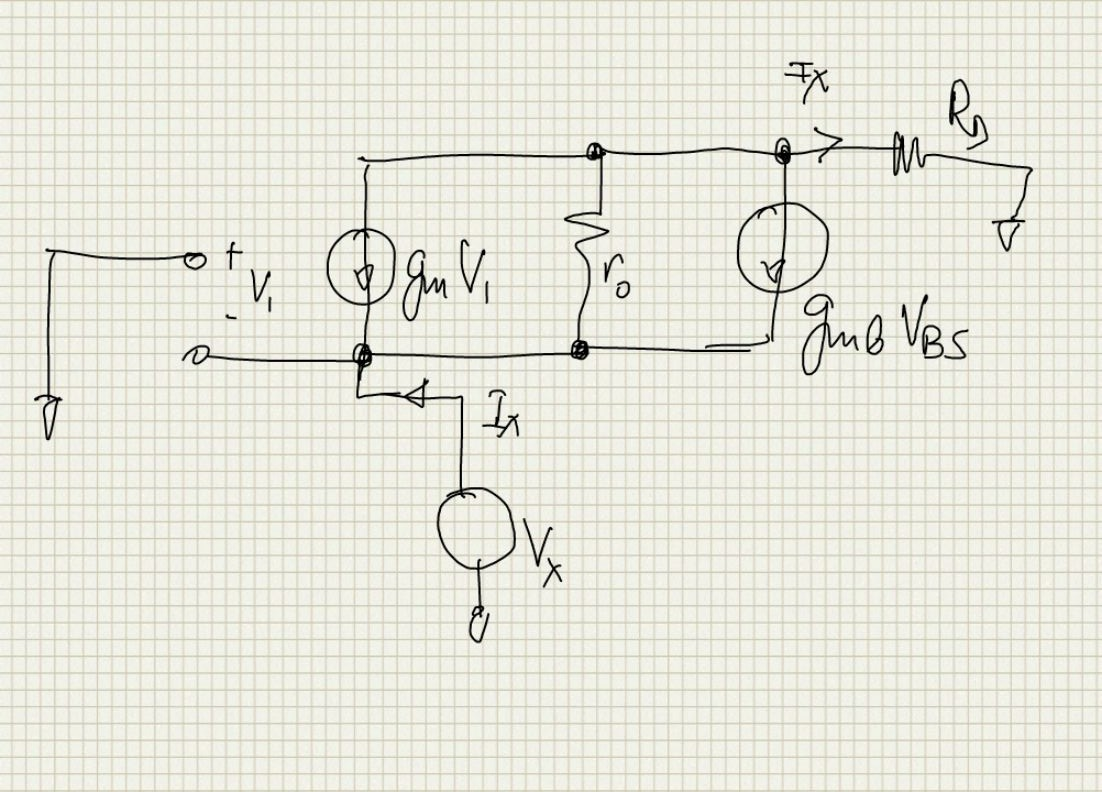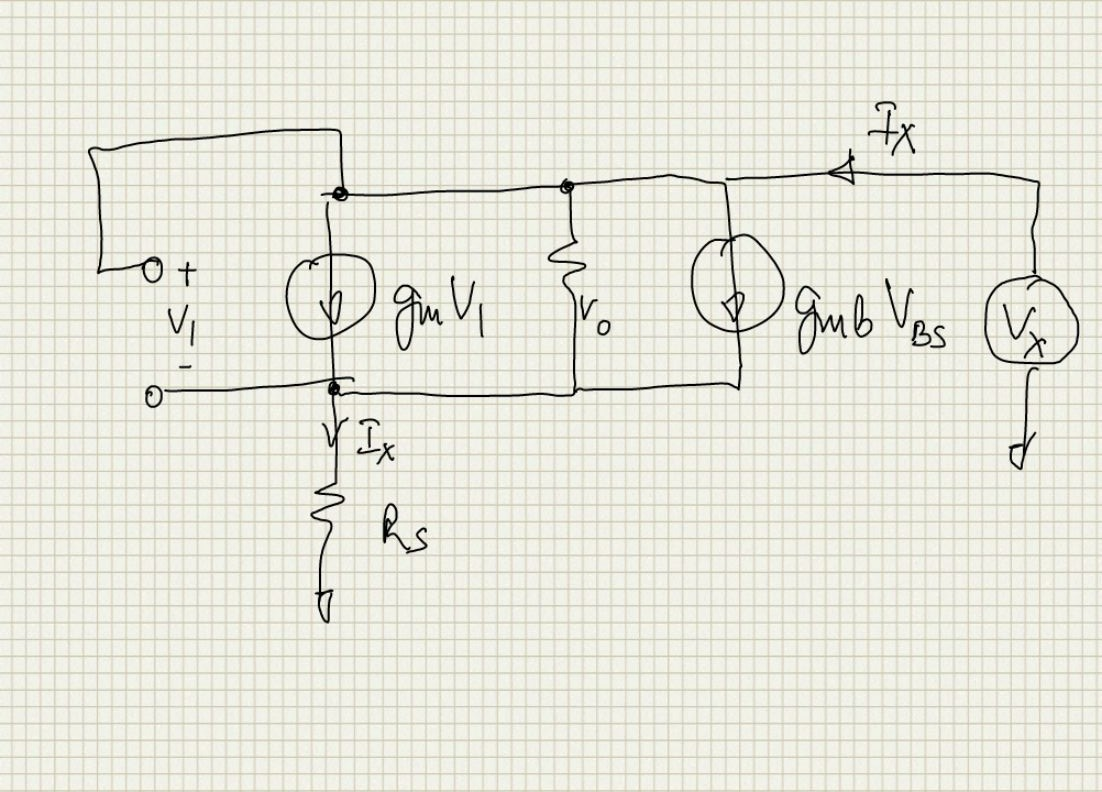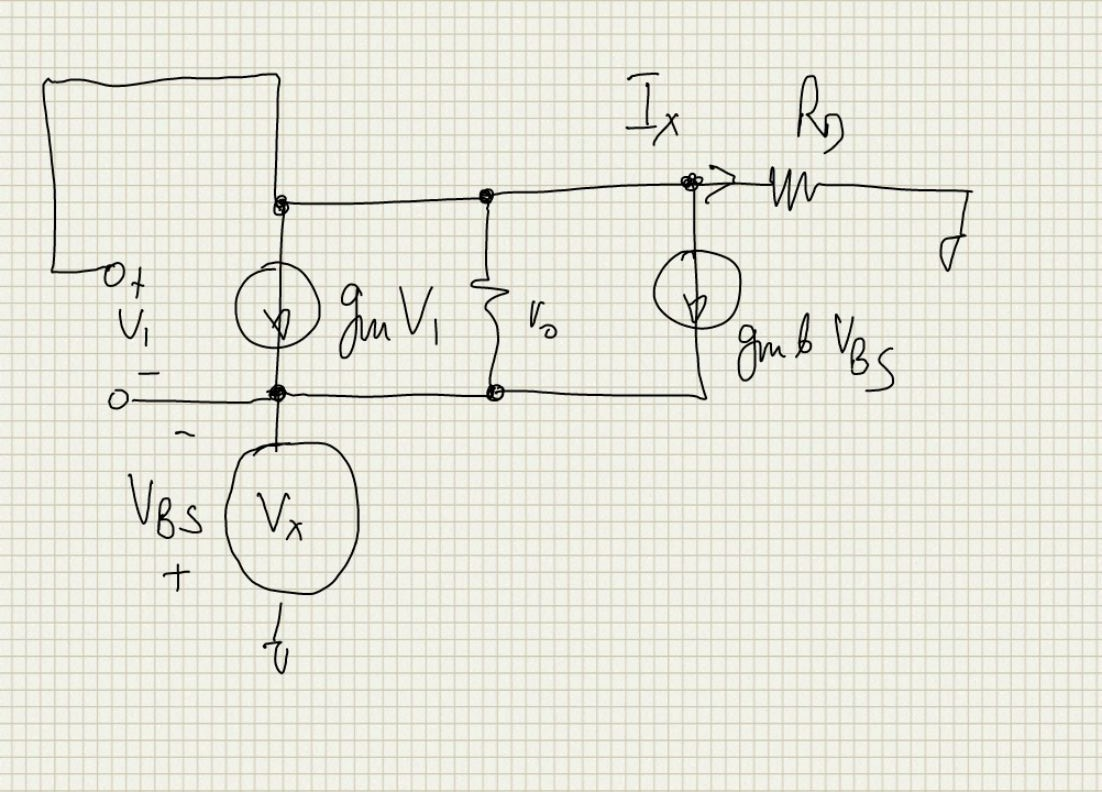# MOS Impedances# Amplification mode [top]

Here we derive the impedances as seen looking into the terminals of a MOS device connected in amplification mode. The setup is as follows:## Gate Impedance [top]

The DC impedance looking into the gate is practically infinite since the gate leakage is very small

## Drain Impedance [top]

The small signal circuit to derive the drain impedance is as follows:We can write the KVL from the test source to ground through Rs and from gate to ground through Rs we have the following 3 equations:
`\$\$(I_1-g_mV_1-g_{mb}V_{BS})r_o+I_xR_S=V_x\$\$`

`\$\$V_1+I_xR_S=0\$\$`

`\$\$V_{BS} = -I_xR_S\$\$`

Eliminating `\$V_1\$` and `\$V_{BS}\$` and solving for `\$V_x/I_x\$` we have
`\$\$Z_D = r_o+R_S(1+r_o(g_m+g_{mb}))\$\$`

### Special Cases: [top]

1. If `\$g_m≫g_{mb}\$` and `\$r_og_m≫1\$` and `\$R_Sg_m≫1\$` then the expression simplifies to:
`\$\$Z_D = r_oR_Sg_m\$\$`
2. If `\$R_S=0\$` then
`\$\$Z_D = r_o\$\$`

## Source Impedance [top]

The small signal circuit to derive the source impedance is as follows:Again writing the KVL from `\$V_x\$` to the gate and to the drain we have the following equations:
`\$\$V_x = -V_1\$\$`

`\$\$V_x=-V_{BS}\$\$`

`\$\$(I_x+g_mV_1+g_{mb}V_{BS})r_o+I_xR_D=V_x\$\$`

Eliminating `\$V_1\$` and `\$V_{BS}\$` and solving for `\$V_x/I_x\$` we have
`\$\$Z_S = {r_o+R_D}/{1+r_o(g_m+g_{mb})}\$\$`

### Special Cases [top]

If `\$R_D=0\$` then
`\$\$Z_S = r_o∥{1/{g_m+g_{mb}} }\$\$`

# Diode Connected Mode [top]

Here we derive the impedances as seen looking into the terminals of a MOS device connected as a diode. The setup is as follows:## Gate-Drain Impedance [top]

The small signal circuit to derive the Gate-Drain impedance is as follows:Writing the KVL through the `\$V_1\$` loop and through the `\$r_o\$` we have the following equations:
`\$\$V_x=I_xR_S+V_1\$\$`

`\$\$V_{BS}=-I_xR_S\$\$`

`\$\$(I_x-g_mV_1-g_{mb}V_{BS})r_o+I_xR_S=V_x\$\$`

Eliminating `\$V_1\$` and `\$V_{BS}\$` and solving for `\$V_x/I_x\$` we have
`\$\$Z_D = {r_o+R_S(1+r_o(g_m+g_{mb}))}/{1+g_mr_o}\$\$`

### Special Cases [top]

1. If `\$R_S=0\$` then the expression reduces to
`\$\$Z_D=r_o∥1/g_m\$\$`
2. For `\$r_o→∞\$` and `\$g_m ≫g_{mb}\$` then the expression reduces to:
`\$\$Z_D=1/g_m+R_S\$\$`

## Source Impedance [top]

The small signal circuit to derive the Source impedance is as follows:Writing the KVL through the `\$V_1\$` loop and through the `\$r_o\$` we have the following equations:
`\$\$V_x+V_1=I_xR_D\$\$`

`\$\$V_{BS}=-V_x\$\$`

`\$\$(I_x+g_mV_1+g_{mb}V_{BS})r_o+I_xR_D=V_x\$\$`

Eliminating `\$V_1\$` and `\$V_{BS}\$` and solving for `\$V_x/I_x\$` we have
`\$\$Z_S = {r_o+R_D+r_og_mR_D}/{1+(g_m+g_{mb})r_o}\$\$`

### Special Cases [top]

1. If `\$R_D=0\$` then the expression reduces to
`\$\$Z_S=r_o∥1/{g_m+g_{mb}}\$\$`
2. For `\$r_o→∞\$` and `\$g_m ≫g_{mb}\$` then the expression reduces to:
`\$\$Z_D=1/g_m+R_D\$\$`

# Reference Books [top]

• Design of Analog CMOS Integrated Circuits